Srivastava, P., and Hasan, S. H. (2011). "Biomass of Mucor heimalis for the biosorption of cadmium from aqueous solutions: Equlibrium and kinetic studies," BioRes. 6(4), 3656-3675.

#### Abstract

The present study deals with the utilization of a cellulosic material, i.e. fungal biomass of Mucor heimalis, for the removal of cadmium from aqueous solution in a batch system. Effects of various parameters such as pH, biomass dosage, contact time, and initial metal concentrations were investigated. The sorption of cadmium followed pseudo-second-order rate kinetics (R2=0.998). Intraparticle diffusion was found not to be the sole rate-controlling step. Thermodynamic studies revealed that the sorption of cadmium was feasible, spontaneous, and exothermic. Various isotherm models viz. Langmuir, Freundlich, Redlich–Peterson, Dubinin-Radushkevich, and Temkin isotherms were applied. The Langmuir and Redlich–Peterson models were found to be in good agreement with experimental data with high R2, low RMSE, and low χ2 values. The Redlich–Peterson isotherm constant g was found to be unity, which implies a good fit to the Langmuir model. The maximum sorption capacity calculated from the Langmuir isotherm was 85.47 mg/g at optimum conditions of pH 6.0, contact time of 35 min, biomass dosage of 1g/L, and temperature of 25 oC.

Biomass of Mucor heimalis for the biosorption of cadmium from aqueous solutions: Equilibrium and kinetic studies

Preeti Srivastava and Syed Hadi Hasan *

The present study deals with the utilization of a cellulosic material, i.e. fungal biomass of Mucor heimalis, for the removal of cadmium from aqueous solution in a batch system. Effects of various parameters such as pH, biomass dosage, contact time, and initial metal concentrations were investigated. The sorption of cadmium followed pseudo-second-order rate kinetics (R2=0.998). Intraparticle diffusion was found not to be the sole rate-controlling step. Thermodynamic studies revealed that the sorption of cadmium was feasible, spontaneous, and exothermic. Various isotherm models viz. Langmuir, Freundlich, Redlich–Peterson, Dubinin-Radushkevich, and Temkin isotherms were applied. The Langmuir and Redlich–Peterson models were found to be in good agreement with experimental data with high R2, low RMSE, and low χ2 values. The Redlich–Peterson isotherm constant g was found to be unity, which implies a good fit to the Langmuir model. The maximum sorption capacity calculated from the Langmuir isotherm was 85.47 mg/g at optimum conditions of pH 6.0, contact time of 35 min, biomass dosage of 1g/L, and temperature of 25 oC.

Key words: Cadmium; Surface area; Kinetic study; Thermodynamic study; Isotherm

Contact information: Water Pollution Research Laboratory, Department of Applied Chemistry, Institute of Technology, Banaras Hindu University, Varanasi- 221 005, U.P., India.

* Corresponding author: shhasan.apc@itbhu.ac.in

INTRODUCTION

The contamination of aqueous environments by heavy metals has become a worldwide environmental problem. Among the heavy metals, copper, lead, cadmium, mercury, nickel, and zinc have high priority for removal from aqueous environments (Wang and Chen 2009; Ahluwalia and Goyal 2007). Cadmium as a component of metal alloys is highly corrosion resistant, and therefore it is widely used to plate metal parts used in general industrial hardware, automobiles, electronics, marine, and aerospace industries, in addition to its use in nickel-cadmium batteries, etc. Cadmium has been a major focus in wastewater treatment because it is associated with many health hazards. Cadmium accumulates in human beings, causing erythrocyte destruction, nausea, salivation, diarrhea, muscular cramps, renal degradation, chronic pulmonary problems, skeletal deformity, proteinuria, glucosuria, etc. (Dara, 2005). The maximum concentration in drinking water, as recommended by the World Health Organization (WHO) and American Water Works Association (AWWA), is 0.005 mg Cd/L. Thus, it is imperative that cadmium be removed from effluent before being discharged into sewage systems or into the aquatic environment.

The most widely used methods for removing heavy metals from wastewaters include ion-exchange, chemical precipitation, reverse osmosis, evaporation, membrane filtration, and adsorptive biological treatment (Pan et al. 2005). Most of these methods suffer from some drawbacks, such as high capital and operational cost or the disposal of the residual metal sludge, and are not suitable for small-scale industries (Kobya et al. 2005).

Biosorption plays an important role in the elimination of metal ions from aqueous solutions in water pollution control (Veglio and Beolchini, 1997; Ferraz, et al. 2004). The main advantages of this technique are the reusability of biomaterial, low operating cost, improved selectivity for specific metals of interest, removal of heavy metals from effluent irrespective of toxicity, short operation time, and no production of secondary compounds that might be toxic (Mungasavalli et al. 2007). Batch experiments are generally done to measure the effectiveness of adsorption for removing specific adsorbates as well as to determine the maximum adsorption capacity. Different types of biomaterial such as Hylocomium splendens (Sari et al. 2008), Lactarius scrobiculatus (Anayurt et al. 2009), Drepanocladus revolvens (Sari and Tuzen 2009), Xanthoparmelia conspersa (Tuzen et al. 2009), Amanita rubescens (Sari and Tuzen et al. 2009), Fucus vesiculosus (Mata et al. 2009), Brown algae (Liu et al. 2009), Ralastonia eutropha & Saccharomyces cerevisiae (Fereidouni et al. 2009)wheat straw (Dang et al. 2009), and mushrooms (Vimala and Das 2009) have been used for the removal of cadmium using batch mode of sorption experiments.

The objective of the present work is to investigate the biosorption potential of Mucor hemalis biomass for the removal of cadmium from aqueous solution. Optimum biosorption conditions were determined as a function of pH, biomass dosage, contact time and initial metal concentrations. Kinetics and thermodynamics studies were also carried out. In addition to this, different models such as Langmuir, Freundlich, Redlich-Peterson (RP), Dubinin-Radushkevich (D-R), and Temkin isotherms were used to describe equilibrium isotherms.

Experimental

Preparation of Microorganism

The strain used in this study, Mucor heimalis (NCIM 873), was obtained from National Chemical Laboratory Pune, India. The culture was routinely maintained at 4 oC on potato dextrose agar medium slant (200 g of peeled potatoes; 20g of dextrose 0.1 g of yeast extract, and 20 g of agar) (Himedia Chemicals, Mumbai) and aerobically cultivated in potato dextrose broth. The flasks were incubated at 30 oC in a rotatory shaker (Orbital shaker) (Scigenics, ORBITEK, Chennai, India) at 120 rpm for 48 h. The growing cells from the culture broth were separated from the liquid by filtration and washed several times with double-distilled water.

The wet cell biomass was dried for 24 h. at 60 °C in an oven. Dried cells were powdered by blender in uniform size and used for sorption experiment.

Preparation of Standards and Reagents

All chemicals and reagents used were of analytical grade and were used without further purification (purchased from E. Merck, India Ltd., Mumbai, India). Stock solutions of Cd(II) 1000 mg/L were prepared from 2.74g of Cd(NO3)2.4 H2O in 1000 mL of de-ionized, double distilled water containing a few drops of concentrated HNO3 to prevent the precipitation of Cd(II) by hydrolysis. Required initial concentration of Cd(II) samples was prepared by appropriate dilution of the above stock Cd(II) standard solution. Standards for calibration of AAS for Cd(II) were prepared from standard solution of cadmium purchased from E. Merck, India Ltd. Mumbai, India.

Physicochemical Characterization of the Biomass

Textural characteristics were determined by nitrogen adsorption at 77.15K to determine the specific surface area of the biomass using a Micromeritics surface area analyzer (Model ASAP 2020, USA) by the Brunauer-Emmett-Teller (BET) method and Barrett-Joyner-Hanlenda (BJH) method. Particle density was determined with the help of specific gravity bottles methods based on the Archimedes’ principle. Bulk density of biomass was measured with Archimedean immersion methods. Particle size was analyzed by sieve analysis with the help of 150 No. BSS sieve. Humidity of biomass was analyzed by psychrometer (DTH 31 digital psychrometer).

The point of zero charge (pHZPC) of the biomass was determined by the solid addition method (Balistrieri and Murray et al. 1981; Mall et al. 2008). For this purpose a series of 100 mL of Erlenmeyer flasks 45 mL of standard solution of 0.1 M KNO3 (A.R. grade Qualigens chemical) was transferred. The pHs of the solution (pHi) were approximately adjusted from 1.0 to 12 by adding either 0.1N HNO3 or NaOH (Qualigens Chemical, Mumbai). The total volume of the solution in each flask was made exactly to 50 mL by adding the 0.1 M KNO3 solution of the same strength. The pHi of the solutions was then accurately noted, and 0.1 g of biosorbent was added to each flask, which were securely capped immediately. The suspensions were then manually shaken and allowed to equilibrate for 48h with intermittent manual shaking. The final pH values of the supernatant liquid (pHf) were noted. The difference between the initial and final pH (∆pH) values (∆pH=pHi−pHy-axis) was plotted against the initial pH(x-axis). was plotted against the pHi. The point of intersection of the resulting curve at which pHi gave the pHZPC.

Batch Sorption Experiments

Biosorption experiments were performed in 250 mL conical flasks previously rinsed with HNO3 in order to remove any metal that remained unabsorbed on the glass wall. The pH of the metal solutions in the conical flask was initially adjusted to desired values by using 0.1 M/HNO3/NaOH (Qualigens Chemical, Mumbai); the sorbents (free biomass and immobilized biomass) were added to each flask and were agitated on the shaker until the equilibrium was reached. The sorbent (free biomass and immobilized biomass), separated by centrifugation/ filtration at 15000 rpm for five minutes, was analyzed for remaining Cd(II) concentration in the sample. The biosorption capacity of the metal ion was calculated by the equation,

q = (Co – Ce) XV/M (1)

where q is the metal uptake (mg/g), V is the volume (L), W is the amount of biomass (g) and Co and Ce are the initial and equilibrium metal concentrations (mg/L), respectively. All experiments in this work were conducted in triplicate, and concordant values were taken.

Analysis of Cd(II) in Aqueous Solution

The analysis of cadmium in sample solutions was done using an Atomic Absorption Spectrophotometer, AAS (Shimadzu AA-6300, Japan). The hollow cathode lamp was used as light source and was set at 228.8 nm wavelength for Cd(II), using 10 mA lamp current and 0.7 nm slit width, and with deuterium lamp for background correction. In order to generate the flame, instrument-grade (98%) acetylene was delivered at 4.0 L/min at a pressure of 0.9 kg/cm2, together with compressed air supplied at 17.5 L/min flow rate and 3.5 kg/cm2 gas pressure. The instrument was calibrated from 0.1 to 10.0 mg/L for Cd(II). Other range samples were diluted until results within the calibration range were obtained for the metal ion.

Results and Discussion

Characterization of Biosorbent

The surface area, pore volume, pore size, and other physicochemical parameters were analyzed, and calculated results are given in Table 1. The surface area of Mucor heimalis at single point (PPo = 0.321904273) was 0.3129 m2/g. The BET surface area was found to be 0.2057 m2/g, whereas BJH adsorption/desorption surface area of pores were 0.0529 m2/g. The single point total pore volume at P/Po = 0.986989152 was 0.5224377 cm3/g. The BJH adsorption/desorption average pore radius was 22.124553 ÅThis biosorbent can be placed in the category of mesoporous (2<d<50 nm), as given by the International Union of Pure and Applied Chemistry (IUPAC) manual for pore size (IUPAC Manual 1982; Mall et al. 2006). Table 1 shows that the bulk density was 0.124 g/cm3, whereas particle density was 0.22 g/cm3. Porosity was 0.437. Particle size for the biomass was analyzed by single sieve (150 No. BSS), and the particle size was 100 µm. This was helpful in keeping the sorption process uniform. Moisture content was 2.12%.

To understand the adsorption mechanism, it is necessary to determine the point of zero charge (pHZPC) of the sorbent. Sorption of cations is favored at pH > pHZPC, while the sorption of anions is favored at pH < pHZPC. The specific adsorption of cations shifts pHZPC towards lower values, whereas the specific adsorption of anions shifts pHZPC towards higher values. In simple term, the value of the pH necessary to affect a net zero charge on a solid surface in the absence of specific biosorption is called the point of zero charge, pHZPC. This is the convenient index of a surface when the charge becomes either positive or negative as a function of pH. Both positive and negative ∆pH values recorded for the biomass are plotted against the initial pH, pHi, and values to yield the pHZPC at the pH value where ∆pH was 0.

Figure 1 represents the point of zero charge of MHB. The figure reveals that ∆pH became negative after initial pH of 3.0. Below this pHZPC, ∆pH had a positive value. Extrapolation of the plot of ∆pH versus pHi to the ∆pH0 lines indicated that the pHZPC of the dead biomass is 3.0The surface of the biomass becomes negative charged at pH extending from these pHZPC up to 10. And biomass surface acquires positive charge below these pHZPC values.

Analysis of all the physiochemical characterization results indicates that dead biomass of Mucor heimalis was potentially suitable for biosorption of cadmium.

Table 1. Physicochemical Characterization of Mucor heimalis Biomass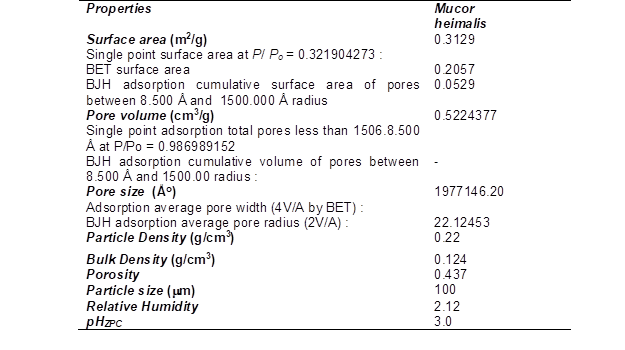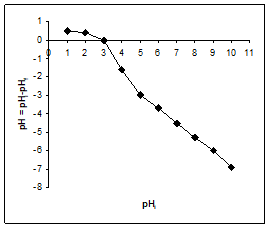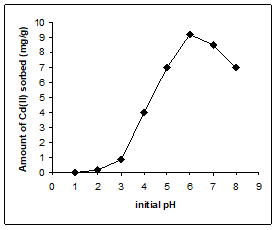Fig. 1. Point of zero charge of biomass          Fig. 2. Effect of pH: Co=10mg/L, T=25oC, M=1g/L

Effect of Initial pH

The interaction between the metal ions and the functional groups present on the biomass depends on the nature of the biosorbent as well as the aqueous chemistry of the metal ions. The pH is a very important parameter, as it strongly affects metal biosorption, surface charge of the biosorbent, ionic mobility, degree of ionization, and the speciation of biosorbate species (Cieslak-Golonka 1991; Tan et al. 2003; Zubair et al. 2008). Cadmium biosorption was investigated by varying the pH from 1.0 to 8.0 (a pH below the onset of metal hydrolysis and precipitation (Mohan et al. 2007; Lee and Davis 2001). The experiment was performed in Erlenmeyer flasks containing 50 mL of an initial 10 mg/L cadmium solution at 25°C temperature, and 1g/L biomass dose. The result thus obtained (Fig. 2) indicated that uptake of cadmium was very low at pH below 2.0. The optimum pH for maximum uptake of 9.2 mg/g for Cd(II) was achieved at pH 6.0. It is also clear from figure that an increase in uptake of Cd(II) was observed with increasing pH from 3 to 6.0. At pH above 6.0, biosorption capacity of the biomass was decreased. This can be explained based on the fact that at low pH the functional groups on the surface of the biosorbent got protonated, which restricts the approach of metal cations as a result of the repulsive force, whereas as the pH increases, more of the functional group would be in dissociates form, carrying negative charges that are attracted to the metal ions. This description is supported by the pHZPC value of biomass of M. heimalis, indicating that surface became negative above 3.0, which facilitated the attraction of positively charged anions (Cd2+) to the negative biomass surface. The adsorption decreased above pH 6 because progressively more M(OH)n is formed, and that such species do not adsorb well.

Effect of Biomass Dose

Experiments were performed at various biomass doses from 1 to 2.5 g/L in 250 mL conical flasks containing 50 mL of initial concentration of 10 mg/L at optimized pH 6.0 and 2 5oC temperature (figure not given). The percentage removal of cadmium was found to be increased from 92% to 99.6 % with the increase of biosorbent dose from 1.0 to 2.5 g/L. Increase in the adsorption with the increase in the adsorbent dosage can be attributed to increased sorbent surface area of mesopores and the availability of more sorption sites.

Effect of Contact time and Initial Metal Concentration

The contact time and initial metal concentration are key parameters for water/ wastewater treatment. A short contact time to attain the saturation stage and the maximum amount of the metal ion sorbed at equilibrium indicate the rapid transport of metal ion from the bulk to the outer and inner surfaces of the biosorbent. Thus, the period of equilibration is an important factor by which to judge the feasibility of a biosorbent for its use in water quality control. In addition to this, the contact time also governs the development of charges at the solid – solution interface. For this purpose experiments were performed at different concentration from 10 to 50 mg/L at biomass dosage 1g/ L and at optimized pH 6.0 and 25 oC temperature. For each metal concentration the contact time was 60 min. and the samples were taken out for analysis at 5 minute intervals. The results thus obtained are presented in Fig. 3. The result showed that equilibrium was established within a contact time of 35 min. for sorption of cadmium and that sorption did not change with further increase of time up to 60 min. The equilibrium time was independent of the concentration of the metal ion solution. The experimental result also showed that the sorption process in all the cases occurred in two phases, i.e. a rapid initial sorption rate followed by a slower rate in later stages. This means that uptake of metal ions was rapid in the initial stages and gradually decreased with the passage of time until equilibrium was approached in each case. This happens because initially the sorption sites are available, the metal ions interact easily with the sites and hence a higher rate of sorption is observed. Further, the driving force for sorption, i.e. the concentration difference between the bulk solution and the solid liquid interface, is higher in initial stages and this results in a higher sorption slowed due to slower diffusion of solute into the interior of the sorbent (Amarasinghe and Williams 2007).

The results further indicated that the initial metal ion concentration had an impact on the process of sorption, with the higher concentration resulting in high solute uptake. From the figure it was found that the uptake capacities were increased from 9.2 to 43.2 mg/g with the increase of initial metal ion concentration from 10 to 50 mg/L. This can be explained on the basis that at lower initial metal ion concentrations, the ratio of the initial moles of metal ion to the available surface area was low, so the sorption becomes independent of the initial concentration. However, at higher concentrations, the sites available for sorption became less in comparison to the moles of metal ion present in solution, and hence the removal of metal ion is strongly dependent upon the initial solute concentration.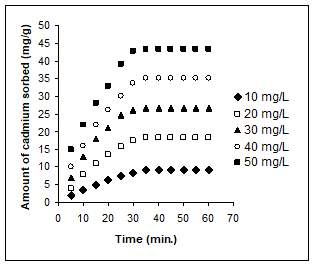Fig. 3. Effect of Contact time and initial metal concentration: Co=10-50mg/L, T=25oC, M=1g/L, pH 6.0

Kinetics is one of the major approaches used to evaluate biosorption dynamics. Kinetic constants can be used to optimize the residence time of a biosorption process. In order to examine the controlling mechanism of the biosorption process, various kinetic models were used to test the experimental data. In order to obtain the rate constants and order of sorption reaction, pseudo-first-order and pseudo-second-order kinetics model were applied to the kinetic data obtained at 25C, at an optimize pH of 6, and initial Cd(II) concentration of 10 mg/L at 1g/L biomass dose and at 5 min interval up to 60 min.

Pseudo-First-Order Model

Different kinetic models have been used to describe the experimental data of heavy metals adsorption on biomass (Lagergren 1898; Ho and Mackay et al. 1999). Pseudo first order Kinetic model of Lagergren (1898) is based on the solid capacity for sorption analysis and expressed as follows,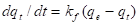(2)

where qt is the amount of adsorbate adsorbed at time (mg/g), kf is the rate constant of pseudo-first-order kinetics (min−1) and t is the time (min). The integration of Eq. (3) with the initial condition, qt =0 at = 0 leads to the pseudo first-order rate equation: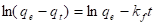(3)

A straight line of ln(qe −qt) versus suggests the applicability of this kinetic model. Pseudo first order rate constant (kf) (1/min) can be determined from the slope of the plot. This equation is, however, valid only for the initial adsorption period.

Pseudo-Second-Order Model

The pseudo-second order reaction kinetic model based on the sorption equilibrium capacity can be expressed as (Ho 2006),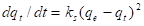(4)

where kS is the pseudo-second-order rate constant (g/mg min). Integrating Eq. (4) and noting that qt =0 at = 0, the following equation is obtained: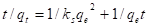(5)

The plot t/qt versus t should give a straight line if second-order kinetics are applicable, and qe and ks can be determined from the slope and intercept of the plot, respectively. The initial sorption rate, (mg/g min), as t→0 can be defined as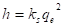(6)

The straight line plot for the pseudo first order sorption kinetic model between ln(qe−qt) vs. t was plotted (Fig. 4) for sorption of cadmium. The value of the rate constant calculated from the slope of plot with the correlation coefficient was 0.086 min-1 and 0.945. The linear plot of pseudo second order kinetic model was also plotted between t/qt vs. t, and sorption capacity and pseudo second order rate constants qe and ks were calculated from the slope and intercept of the plot (Fig. 5). The pseudo second order kinetic constant ks and sorption capacity qe were 0.000807 g/mg min and 23.31 mg/g respectively. A high coefficient of determination, i.e 0.998, was obtained for the pseudo second order kinetic model, and these results were good in comparison to pseudo first order model, indicating that process of uptake of cadmium followed pseudo second order rate expression. In addition to this initial sorption rate (h) were also calculated by using Eq. 6. The initial sorption rate was 0.438 mg/gmin.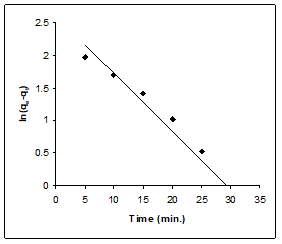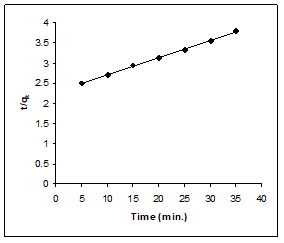Fig. 4. Pseudo first order plot                              Fig. 5. Pseudo Second Order model plot

Co= 10 mg/L, T=25oC, M=1g/L, pH=6                Co= 10 mg/L, T=25oC, M=1g/L, pH=6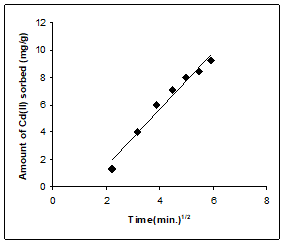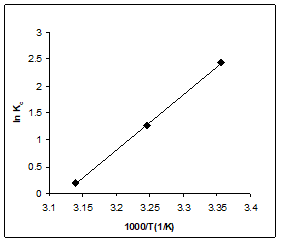Fig. 6. Intraparticle diffusion coefficient            Fig. 7. Van’t Hoff Plot

Co= 10 mg/L, T=25oC, M=1g/L, pH=6

Intraparticle Diffusion Study

Bangham’s equation

Kinetic data were further used to help understand the slow step occurring in the present adsorption systems using Bangham’s equation (Aharoni 1979),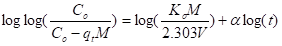(7)

where α (<1) and Ko are constants. The log log (Co/(Co − qtM)) vs. log(t) plot (not given) did not yield linear curves, showing that the diffusion of cadmium into the pores of Mucor heimalis is not the only rate-controlling step (Tutem et al. 1998). Values of the Bangham parameters α and Ko were 0.689 and 3.43 mL/g/L with a coefficient of determination of 0.946.

Intraparticle Diffusion Model

The possibility of intra-particle diffusion was explored by using the intra-particle diffusion model,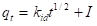(8)

where kid is the intra-particle diffusion rate constant. According to Eq. (8), a plot of qt versus t1/2 should be a straight line with a slope kid and intercept when adsorption mechanism follows the intra-particle diffusion process. It is clear from figure (Fig. 6) that the plot was linear within certain range, but the trend of the data did not pass from the origin. The value of kid was 2.07 mg/g min with correlation coefficient 0.963.The value of I, i.e. 2.67 mg/g, gives an idea about the thickness of the boundary layer, i.e. the larger the intercept, the greater is the boundary layer effect (Kannan and Sundaram 2001). The deviation of the straight lines from the origin may be due to the difference in the rate of mass transfer in the initial and final stages of adsorption. Further, such deviation of the straight lines from the origin indicates that the pore diffusion is not the sole rate-controlling step (Mall 2006).

Thermodynamic Study

Thermodynamic considerations of a biosorption process are necessary to conclude whether the process is spontaneous or not. The Gibbs free energy change, G, is an indication of spontaneity of a chemical reaction and therefore is an important criterion for spontaneity. Also, both energy and entropy factors must be considered in order to determine the Gibbs free energy of the process. Reactions occur spontaneously at a given temperature if G is a negative quantity. The free energy of a biosorption reaction, considering the biosorption equilibrium constant Kc is given by the following equation,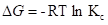(9)

where G is the standard free energy change (kJ/mol), the universal gas constant, 8.314 J/mol K, and is absolute temperature (K). Kc is the equilibrium constant, which is calculated from following equation,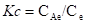(10)

where CAe is the equilibrium concentration of metal ion on the sorbent (mg/L) and Ce is the equilibrium concentration of metal ion in bulk solution (mg/L). Kc may be expressed in terms of ∆H (kJ/mol) and ∆S (J/mol/K) as a function of temperature. It is known that ∆G is the function of change of enthalpy of sorption (∆H, kJ/mol) as well as change in entropy (∆S, J/mol/K):

G = ∆– TS (11)

If Eq. 9 is inserted into Eq. 11, it becomes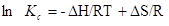(12)

Change of enthalpy ∆H (kJ/mol) and change of entropy ∆S (J/mol/K) of sorption can be calculated from the slope and intercept of the plot of ln Kc and 1/T (Van’t Hoff plot).

Thermodynamic parameters viz. Gibb’s free energy change of sorption (G) (KJ/mol), enthalpy change (H) (kJ/mol), and standard entropy change (S) (J/mol/ K) of sorption of cadmium can be evaluated on the temperature range of 25 to 45 ºC on the biomass of M. heimalis. For this purpose, the van’t Hoff plot was drawn for sorption of cadmium between ln Kc vs. 1/T and results are shown in Fig.7. The van’t Hoff plot gave a straight line. The thermodynamic parameter, i.e. the free energy change ∆G, for any feasible process, should be negative. It is clear from the result that the free energy change (∆G) was negative, which indicates that the process of sorption was spontaneous. The sorptive forces were quite strong to overcome the potential energy barrier. The negative value of ∆G for cadmium ion was decreased from -6.04, -3.24, and -0.530 kJ/mol with increase of temperature from 25 to 45 oC. This indicated that the feasibility of the biosorption process decreased at higher temperatures. It is also evident from the result that the enthalpy change (∆H) was negative (-86.207 kJ/mol). This also indicate that the biosorption was accompanied by the evolution of heat, which means that the sorption process were exothermic in nature. It is also evident from the result that entropy change was also negative (269.14 J/mol). The negative value of ∆S reflects the affinity of the M. heimalis for cadmium and suggests some structural changes in cadmium and M. heimalis interaction. In addition to this, negative value of ∆S shows increasing randomness in the system during the biosorption of cadmium ions onto M. heimalis.

Sorption Isotherms

Models have an important role in technology transfer from a laboratory to the pilot plant scale. An appropriate model can help in understanding process mechanisms, analyze experimental data, predict answer to operational conditions, and optimize processes. The Langmuir, Freundlich, Redlich-Peterson (RP), Dubinin-Radushkevich (D-R), and Temkin isotherm models were used in the present work. These models are simple, well established, have physical meaning, and are easily interpretable. For this purpose experiments were performed by varying initial metal ion concentrations from 10 to 50 mg/L for Cd(II) at 25oC temperatures, 1g/L of biomass dose and pH 6.0. In addition to this, the better fit of model was checked by correlation coefficient (r2), residual root mean square error (RMSE), and Chi square (χ2) tests.

Langmuir Isotherm

The Langmuir model (Langmuir 1916) describes monolayer biosorption onto a surface with a finite number of identical binding sites and was derived from gas-phase adsorption onto a homogeneous surface of glass and metals. It is used to estimate the maximum metal sorption values at saturation that could not be reached in the experiment. The non- linear form of Langmuir isotherm model is presented as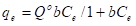(13)

The linear expression of the this isotherm can be written as,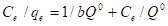(14)

where qe and Qo are the equilibrium and monolayer biosorption capacities of the sorbent (mg/g), respectively, Ce is the residual metal concentration at equilibrium (mg/L), and b is the biosorption equilibrium constant (L/mg ) related to the free energy of biosorption. Qo indicates a practical limiting biosorption capacity when all binding sites are occupied by heavy metal, and it assists in the comparison of biosorption performance.

Freundlich Isotherm

The empirical Freundlich isotherm (Freundlich 1906) based on sorption on a heterogeneous surface, can be derived assuming a logarithmic decrease in the enthalpy of sorption with the increase in the fraction of occupied sites and is given by,

qeKFCe1/n (14)

where KF and 1/are the Freundlich constants characteristics of the system, indicating the sorption capacity and sorption intensity, respectively. Eq. (14) can be linearized in logarithmic form: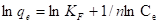(15)

Redlich–Peterson Isotherm

The Redlich–Peterson isotherm (Redlich and Peterson 1959) contains three parameters and incorporates the features of the Langmuir and the Freundlich isotherms. The Redlich–Peterson isotherm has a linear dependence on concentration in the numera-tor and an exponential function in the denominator. It can be described as follows: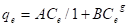(16)

It has three isotherm constants, namely, AB, and (0 < < 1), which characterize the isotherm. Its limiting behavior is summarized as follows: When g equals 1,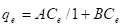(17)

i.e. the Langmuir form results. On the other hand, when the constants and are much greater than unity (Ho, 2004),(18)

i.e. the Freundlich form results. And when g=0,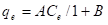(19)

i.e. the Henry’s Law form results.

Equation (13) can be converted to a linear form by taking logarithms: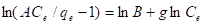(20)

Three isotherm constants, AB, and can be evaluated from the linear plot represented by Eq. (10) using a trial and error procedure, which is applicable to computer operation. Such an approach was developed to determine the isotherm parameters by optimization routine to maximize the coefficient of determination, R2, for a series of values of for the linear regression of ln(Ce) on ln[A(Ce/qe)−1] and to obtain the best value of which yields a maximum ‘optimized’ value of R2 using the solver add-in with Microsoft’s spreadsheet, Microsoft Excel.

The D-R model (Dubinin and Radushkevich, 1947) describes the biosorption nature of the sorbate on the biosorbent and is used to calculate the mean free energy of biosorption. According to this model, the characteristic biosorption curve is related to the porous structure of the biosorbent. Its linearized equation can be represented as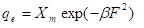(21)

Eq. (21) can be linearized as,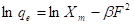(22)

where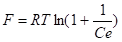is polanyi potential, qe is the amount of sorbate sorbed by the sorbent (mol/g), Xm is the maximum sorption capacity of the sorbent (mol/g), β is the constant related to biosorption energy (mol2/kJ2), R is the universal gas constant [8.314 J/mol/K], T is the absolute temperature (K), and Ce is the concentration at equilibrium (mol/L). The D-R isotherm constants β and Xm were obtained from the slope and intercept of the plot of ln qe against F2. The mean free energy of biosorption (E) – defined as the free energy change when 1 mole of ion is transferred to the surface of the solid from infinity in solution – can be calculated from the β value obtained from: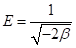(23)

If the magnitude of E is between 8 to 16 kJ mol-1, then the sorption process is supposed to proceed via chemisorption reaction, while for values of E<8kJ/mol, the sorption process is of physical nature.

Temkin Isotherm

The Temkin isotherm equation (Temkin 1940) assumes that the heat of adsorption of all the molecules in the layer decreases linearly with coverage due to adsorbent-adsorbate interactions, and that the adsorption is characterized by a uniform distribution of the binding energies, up to some maximum binding energy. The Temkin isotherm is given as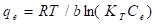(24)

Eq. (24) can be linearized as,

qe = B1ln KT + B1ln Ce (25)

where

B1 = RT/b (26)

A plot of qe versus lnCe enables the determination of the isotherm constants B1 and KT from the slope and the intercept, respectively. KT is the equilibrium binding constant (l/mol) corresponding to the maximum binding energy and constant B1 is related to the heat of adsorption.

In addition to this, the better fit of model was checked by coefficient of determination (R2), residual root mean square error (RMSE), and Chi square (χ2) tests. The residual root mean square error (RMSE) and Chi square (χ2) values were evaluated through the following equations: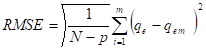(27)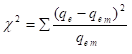(28)

where, qe is the observation from batch experiment, qem is the measured value from the isotherm for corresponding qeN is the number of observation in the experimental design, and p is the number of parameters to be determined. The value of linear coefficient of determination, R2, represents the percentage of variability in the dependent variable that has been explained by the regression line and may vary from 0 to 1. If there is no relationship between the predicted values and the actual values, the coefficient of determination is zero or very low; a perfect fit gives a coefficient of 1.0. On the other hand, in χ2-square test, χ2 is basically the sum of the squares of the differences between the experimental data and data obtained from the models, with each square difference divided by the corresponding data obtained by calculation from the models (Eq. 3.18). Smaller values of RMSE and χ2 and high values of correlation indicate a better fit of model (Tsai and Juang 2000; Ho and Ofomaja 2006).

The Langmuir isotherm plot is shown in Fig. 8. Isotherm constants i.e. Qo and b, were calculated from the slope and intercept of the plot between Ce/qe and Ce. The data thus obtained are presented in Table 2 along with R2, RMSE, and χ2 values. The isotherm showed good fit to the experimental data with high correlation coefficient. The maximum sorption capacity (Qo) of 85.47 mg/g was obtained at temperature 25oC. The Langmuir constant b is related with the affinity of the biosorbent for the sorbate. A low value of b i.e. 0.15 was suggestive of high affinity of the biosorbent for cadmium. The biosorbent capacity of this biomass was much greater in comparison to other biosorbents reported in Table 3. Hence M. heimalis seems to be a good biosorbent for this metal ion as it has high Qo and low b values.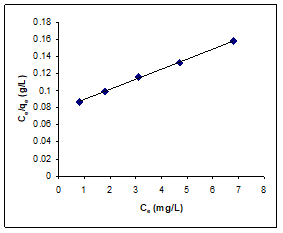Fig. 8. Langmuir isotherm plot: Co= 10 to 50 mg/L, T=25oC, M=1g/L, pH= 6.0

Freundlich constants kF and n were determined from the slope and the intercept of the linearized plots of log qe versus log Ce (figure not given). The data thus obtained are presented in Table 2 along with R2, RMSE and χ2 values. The high values of kF and n showed a high feasibility of Cr(VI), Cd(II), and Pb(II) biosorption. The values of n (intensity of sorption) between 1 and 10 (i.e., 1/n less than 1) represent a favorable sorption. For the present study, the value of n also presented the same trend representing a beneficial sorption. The Freundlich isotherm provides the information about the surface heterogeneity and the exponential distribution of active sites and their energies. It does not predict any saturation of the surface of the sorbent by the sorbate, and hence an infinite surface coverage could be predicted mathematically. The correlation coefficient was found to be 0.994. This indicated that there is good agreement but not a perfect one.

Table 2. Isotherms Constants of Different Isotherm Models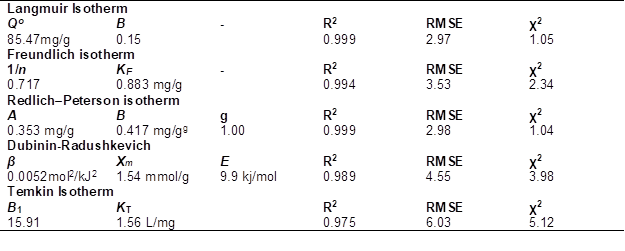Redlich–Peterson isotherm constants AB, and were also calculated from a linear plot ofvs. ln Ce (figure not given) and data are presented in Table 2 along with R2, RMSE and χ2 values. In this case, the value of g is unity, which means the isotherms are approaching the Langmuir isotherm.

Although the Freundlich isotherm provides the information about the surface heterogeneity and the exponential distribution of the active sites and their energies, it does not predict any saturation of the surface of the sorbent by the sorbate. Hence, infinite surface coverage could be predicted mathematically. In contrast, the D–R isotherm relates the heterogeneity of energies close to the adsorbent surface. If a very small sub-region of the sorption surface is chosen and assumed to be approximately by the Langmuir isotherm, the quantity can be related to the mean sorption energy, E, which is the free energy for the transfer of 1 mol of metal ions from the infinity to the surface of the sorbent. D-R isotherm constant β (0.0052) and Xm (1.54 mmol/g or 173.096 mg/g) were obtained from slope and intercept of the plot of ln qe against F2 plot (figure not given), and the values are given in Table 2. The isotherms showed good fit to the experimental data with good coefficients of determination. The estimated value of E was found to be 9.9 kJ/mol, which is the range expected for chemisorptions (8 to 16 kJ/mol). The applicability of this isotherm model to the cadmium sorption shows that there is possibility of heterogeneous energetic distribution of active sites on the surface of the sorbent.

Temkin isotherm constants B1 and KT were determined from the slope and the intercept of the linearized plots of qe versus ln Ce (figure not given). The data thus obtained are presented in Table 2 along with R2, RMSE and χ2 values. The heat of adsorption (B1) is 15.91 and equilibrium binding constant (KT) is 1.56 L/mg.

On the basis of low RMSE and low chi square value and high correlation coefficients (Table 2), it was found that the Langmuir isotherm model and Redlich–Peterson isotherm fitted the result substantially better than the Freundlich isotherm, Dubinin-Radushkevich, and Temkin isotherm models. The applicability of Langmuir and Redlich–Peterson isotherms showed that there were effectively monolayer sorption and a homogeneous distribution of active sites on the surface of the biosorbent. In all the cases, the Temkin isotherm represented the poorest fit of experimental data in comparison to the other isotherm equations. The D-R equation yielded better fit to the experimental data than the Temkin isotherm in the study.

Table 3. Comparison of Cadmium Sorption Capacity of Mucor heimalis with Different Sorbents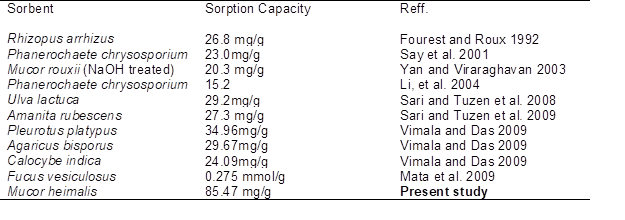CONCLUSIONS

Mucor heimalis biomass has great potential for removing cadmium ions, especially when metal concentration of this metal is low in wastewater streams. The sorption was highly pH dependent. Maximum sorption was obtained at 6.0 pH. The sorption data fitted both in Langmuir and Redlich–Peterson isotherm with high R2, low RMSE, and low χ2 values. The maximum sorption capacity was 85.47mg/g. at 25 oC temperature, 1g/L of biomass dose, and 10 -50 mg/L of initial cadmium concentration range. Thermodynamic studies revealed that sorption was feasible, spontaneous, and exothermic in nature. Sorption followed pseudo second order rate kinetics. In addition, the intraparticle diffusion contant was not the sole rate-controlling step. Based on all results, it can be also concluded that Mucor heimalis can be evaluated as an alternative biosorbent for treatment of cadmium containing wastewater, since Mucor heimalis is low-cost biomass and has a considerably high biosorption capacity.

REFERENCES CITED

Aharoni, C., Sideman, S., and Hoffer, E. (1979). “Desorption of phosphate ions by collodion-coated alumina,” J. Chem. Technol. Biotechnol. 29, 404-412.

Ahluwalia, S. S., and Goyal, D. (2007). “Microbial and plant derived biomass for removal of heavy metals from wastewater,” Bioresour Technol. 98, 2243-2257.

Amarasinghe, B. M. W. P. K., and Williams, R. A. (2007), “Tea waste as low cost adsorbent for the removal of Cu and Pb from waste water,” Chem. Eng. J. 132, 299-309.

Anayurt, R. A., Sari, A., and Tuzen, M. (2009), “Equilibrium, thermodynamic and kinetic studies on biosorption of Pb(II) and Cd(II) from aqueous solution by macrofungus (Lactarius scrobiculatus) biomass,” Chem. Eng. J. 151, 255-261.

Cieslak-Golonka, M. (1991), “Spectroscopy of chromium(VI) species,” Coord. Chem. Rev. 109, 223-249.

Dang, V. B. H., Doan, H. D., Dang-Vu, T., and Lohi, A. (2009). “Equilibrium and kinetics of biosorption of cadmium(II) and copper(II) ions by wheat straw,” Bioresour. Technol. 100, 211-219.

Dara, S. S. (2005). A Textbook of Environmental Chemistry and Pollution Control, S. Chand & Company LD, ISBN 81-219-083-3.

Dubinin, M. M., and Radushkevich, L. V. (1947). “Equation of the characteristic curve of activated charcoal, Proceedings of the Academy of Sciences,” Proceedings of the Academy of Sciences of the USSR, Chemistry Section 55, 331-333.

Fereidouni, M., Daneshi, A., and Younesi, H. (2009). “Biosorption equilibria of binary Cd(II) and Ni(II) systems onto Saccharomyces cerevisiae and Ralstonia eutropha cells: Application of response surface methodology,” J. Hazard. Mater. 168, 1437-1448.

Ferraz, A. I., Tavares, T., and Teixeira, J. A. (2004). “Cr(III) removal and recovery from Saccharomyces Cerevisiae,” Chem. Eng. J. 105, 11-20.

Fourest, E., and Roux, J. C. (1992). “Heavy metal biosorption by fungal mycelial by-products: Mechanism and influence of pH,” Appl. Microbiol. Biotechnol. 37, 399-403.

Freundlich, H. M. F. (1906). “Über die Adsorption in Lösungen,” Zeitschrift für Physikalische Chemie (Leipzig) 57A, 385-470.

Gupta, V. K., (1998). “Equilibrium uptake, sorption dynamics, process development, and column operations for the removal of copper and nickel from aqueous solution and wastewater using activated slag, a low-cost adsorbent,” Ind. Eng. Chem. Res. 37, 192-202.

Ho, Y. S., (2006). “Review of second order models for adsorption systems,” J. Hazard. Mater. 136, 681-689.

Ho, Y. S. (2004). “Selection of optimum sorption isotherm,” Carbon 42, 2115-2116.

Ho,Y. S., and Ofomaja, A. E. (2006), “Biosorption thermodynamics of cadmium on coconut copra meal as biosorbent,” Biochem. Eng. J. 30, 117-123.

IUPAC Manual of Symbols and Terminology of Colloid Surface, Butterworths, London, 1982, p. 1.

Kannan, K., and Sundaram, M. M. (2001). “Kinetics and mechanism of removal of methylene blue by adsorption on various carbons e a comparative study,” Dyes Pigments 51, 25-40.

Kobya, M., Demirbas, E., Senturk, E., and Ince, M. (2005). “Adsorption of heavy metal ions from aqueous solutions by activated carbon prepared from apricot stone,” Bioresour. Technol. 96, 1518-1521.

Lagergren, S. (1898). “Zur Theorie der sogenannten Adsorption geloster Stoffe,” Kungliga Svenska Vetenkapsakademiens, Handlingar 24, 1-39.

Langmuir, I. (1916). “The constitution and fundamental properties of solids and liquids,” J. Am. Chem. Soc. 38, 2221-2295.

Lee, S. M., and Davis, A. P. (2001). “Removal of Cu(II) and Cd(II) from aqueous solution by sea food processing waste sludge,” Water Res. 35, 534-540.

Li, Q., Wu, S., Liu, G., Liao, X., Deng, X., Sun, D., Hu, Y., and Huang, Y. (2004), “Simultaneous biosorption of cadmium (II) and lead (II) ions by pretreated biomass of Phanerochaete chrysosporium,” Sep. Purif. Technol. 34, 135-142.

Liu, Y., Cao, Q., Luo, F., and Chen, J. (2009). “Biosorption of Cd2+, Cu2+, Ni2+ and Zn2+ ions from aqueous solutions by pretreated biomass of brown algae,” J. Hazard. Mater. 163, 931-938.

Mall, I. D., Srivastava, V. C., Kumar, G. V. A., and Mishra, I. M. (2006). “Characterization and utilization of mesoporous fertilizer plant waste carbon for adsorptive removal of dyes from aqueous solution,” Colloid Surf A 278, 175-187.

Mata, Y. N., Blázquez, M. L., Ballester, A., González, F., and Muñoz , J. A. (2009). “Biosorption of cadmium, lead and copper with calcium alginate xerogels and immobilized Fucus vesiculosus,” J. Hazard. Mater. 163, 555-562.

Mohan D., Pittman Jr., C. U., Bricka, M., Smith, F., Yanceyd, B., Mohammad, J., Steele, P. H., Alexandre-Franco, M. F., Gómez-Serrano, V., and Gong, H. (2007). “Sorption of arsenic, cadmium, and lead by chars produced from fast pyrolysis of wood and bark during bio-oil production,” J. Colloid Interf. Sci. 310, 57-73.

Mungasavalli, D. P., Viraraghavan, T., and Jin, Y. C. (2007). “Biosorption of chromium from aqueous solutions by pretreated Aspergillus niger: Batch and column studies,” Colloids Surf. A 301, 214-223.

Pan, X. L., Wang, J. L., and Zhang, D. Y. (2005). “Biosorption of Pb(II) by Pleurotus ostreatus immobilized in calcium alginate gel,” Process Biochem. 40(8), 2799-2803.

Redlich, O., and Peterson, D. L. (1959). “A useful adsorption isotherm,” J. Phys. Chem. 63, 1024.

Sarı, A., and Tuzen, M. (2008). “Biosorption of Pb(II) and Cd(II) from aqueous solution using green alga (Ulva lactuca) biomass,” J. Hazard. Mater. 152, 302-308.

Sari, A., Mendil, D., Tuzen, M., and Soylak, M., (2008), “Biosorption of Cd(II) and Cr(III) from aqueous solution by moss (Hylocomium splendens) biomass: Equilibrium, kinetic and thermodynamic studies,” Chem. Eng. J. 144, 1-9.

Sari, A., and Tuzen, M. (2009), “Removal of mercury(II) from aqueous solution using moss (Drepanocladus revolvens) biomass: Equilibrium, thermodynamic and kinetic studies,” J. Hazard. Mater. 171, 500-507.

Sarı, A., and Tuzen, M. (2009), “Kinetic and equilibrium studies of biosorption of Pb(II) and Cd(II) from aqueous solution by macrofungus (Amanita rubescens) biomass,” J. Hazard. Mater. 164, 1004-1011.

Say, R., Denizli, A., and Arıca, M. Y. (2001). “Biosorption of cadmium(II), lead(II) and copper(II) with the lamentous fungus Phanerochaete chrysosporium,” Bioresour. Technol. 76, 67-70.

Tan, L. H., Chen, J. P., and Ting, Y. P. (2003). “Biosorption of chromium (VI) by algal biomass,” In: Proceedings of the 15th International Biohydrometallurgy Symposium, Athens, Hellas, p. 807.

Temkin, M. I., and Pyzhev, V. (1940). “Kinetics of ammonia synthesis on promoted iron catalysts,” Acta. Physicochim. URSS 12, 327.

Tsai, S. C., and Juang, K. W. (2000). “Comparison of linear and nonlinear forms of isotherm model for strontium sorption on a sodium bentonite,” J. Radioanal. Nucl. Chem. 243, 741-746.

Tutem, E., Apak, R., and Unal, C. F. (1998). “Adsorptive removal of chlorophenols from water by bituminous shale,” Water Res. 32, 2315-2324.

Tuzen, M., Ahmet, S., Durali, M., and Mustafa, S., (2009), “Biosorptive removal of mercury (II) from aqueous solution using lichen (Xanthoparmelia conspersa) biomass: Kinetic and equilibrium studies,” J. Hazard. Mater. 169, 263-270.

Veglio, F., and Beolchini, F. (1997). “Removal of metals by biosorption: A review,” Hydrometallurgy 44, 301-316.

Vimala, R., and Das, N. (2009). “Biosorption of cadmium (II) and lead (II) from aqueous solutions using mushrooms: A comparative study,” J. Hazard. Mater. 168, 376-382.

Wang, J., and Chen, C. (2009). “Biosorbents for heavy metals removal and their future,” Biotechnol. Adv. 27, 195-226.

Yan, G., and Viraraghavan, T. (2003). “Heavy metal removal from aqueous solution by fungus Mucor rouxii,” Water Res. 37, 4468-4496.

Zubair, A., Bhatti, H. N., Hanif, M. A., and Shafqat F. (2008). “Kinetic and equilibrium modeling for Cr(III) and Cr(VI) removal from aqueous solutions by Citrus reticulate waste biomass,” Water Air Soil Pollut. 191, 305-318.

Article submitted: April 13, 2011; Peer review completed: May 13, 2011; Revised version received: July 19, 2011; Further corrections and acceptance: July 29, 2011; Published: July 31, 2011.